﻿ 电磁轨道炮后坐诸元与反后坐装置设计
 舰船科学技术2019, Vol. 41Issue (2): 150-153PDF

Research on recoil data and anti-recoil mechanism design of electromagnetic railgun
QIU Qun-xian, MA Xin-ke, HE Hang, GAO Bo
The 713 Research Institute of CSIC, Zhengzhou 450015, China
Abstract: Anti-electromagnetic-force is produced while projectile is launched in electromagnetic railgun. The existing force will make the recoil assemble move along the opposite direction of projectile. The recoil process can be regarded as two movement periods. Maximum velocity and original recoil displacement can be calculated from free recoil movement period. The maximum recoil displacement obtained from inertia recoil movement period is the direct basis to design the anti-recoil mechanism. According to the recoil differential equation, the results of 0° and 60° elevation were calculated. The research has some reference significance to study anti-recoil mechanism.
Key words: electromagnetic railgun     recoil data     anti-recoil mechanism
0 引　言

1 电磁轨道炮自由后坐时期及自由后坐运动诸元的计算

 $m(v - V) = MV{\text{，}}$ (1)

 $V = \frac{m}{{m + M}}v{\text{。}}$ (2)

 $L = \frac{m}{{m + M}}x{\text{。}}$ (3)

2 电磁轨道炮制动后坐诸元的计算

 $R = {F_Z} + {F_f} + F - Mg\sin \varphi {\text{，}}$ (4)

 $M\frac{{{{\rm d}^2}x}}{{{\rm d}{t^2}}} = M\frac{{{\rm d}v}}{{{\rm d}t}} = {F_{dc}} - R{\text{。}}$ (5)

3 电磁轨道炮自由后坐运动诸元分析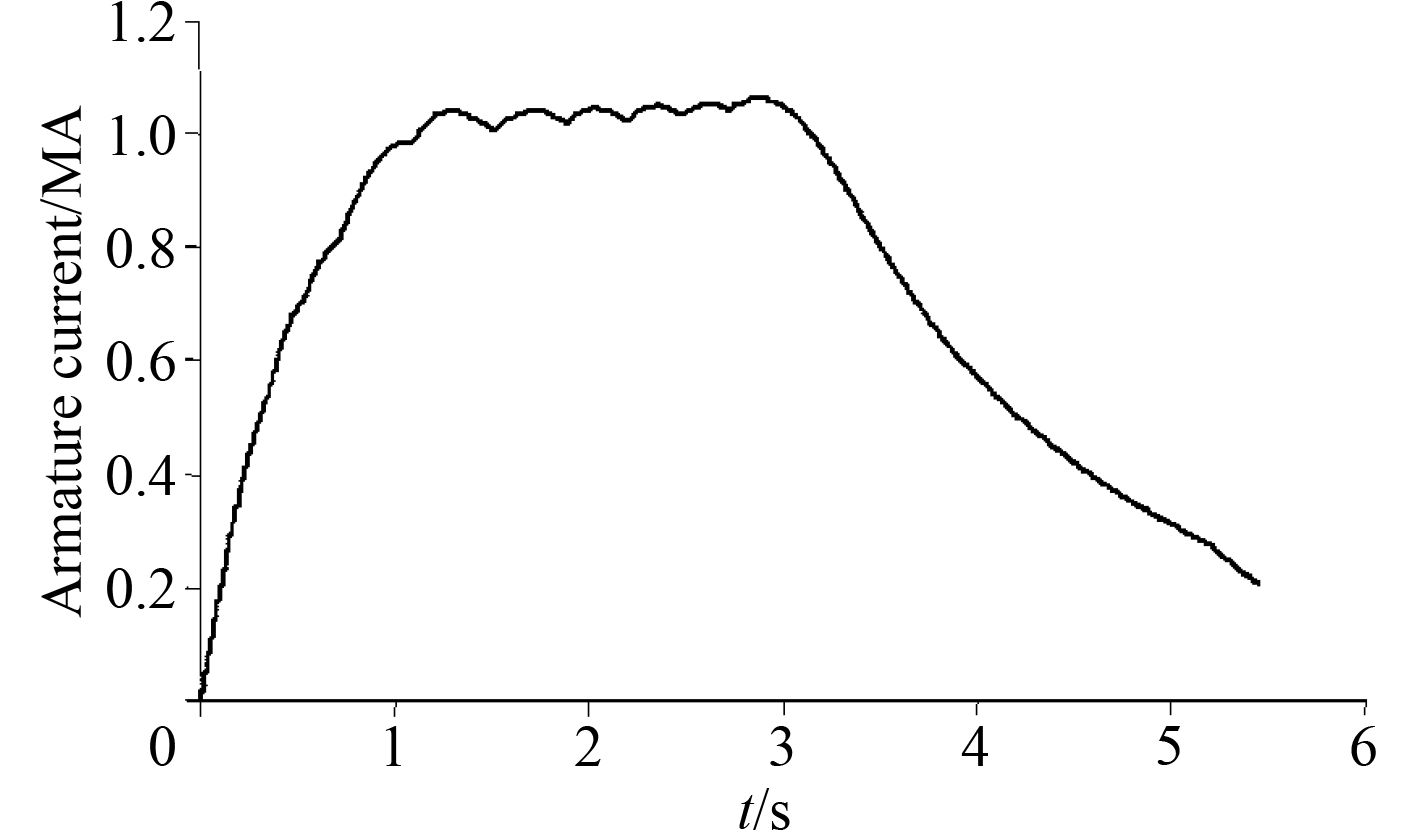图 1 一种电磁轨道炮发射时的电流波形 Fig. 1 One type of shooting current wave for electromagnetic railgun

 $\frac{1}{2}M{V^2} = \mu Mgs{\text{，}}$ (6)
 $s = \frac{1}{{2\mu g}}{V^2}{\text{。}}$ (7)表 1 不同摩擦系数与对应最大后坐行程表 Tab.1 The table of different friction coefficients vs. maximum recoil displacements

4 电磁轨道炮反后坐装置的设计研究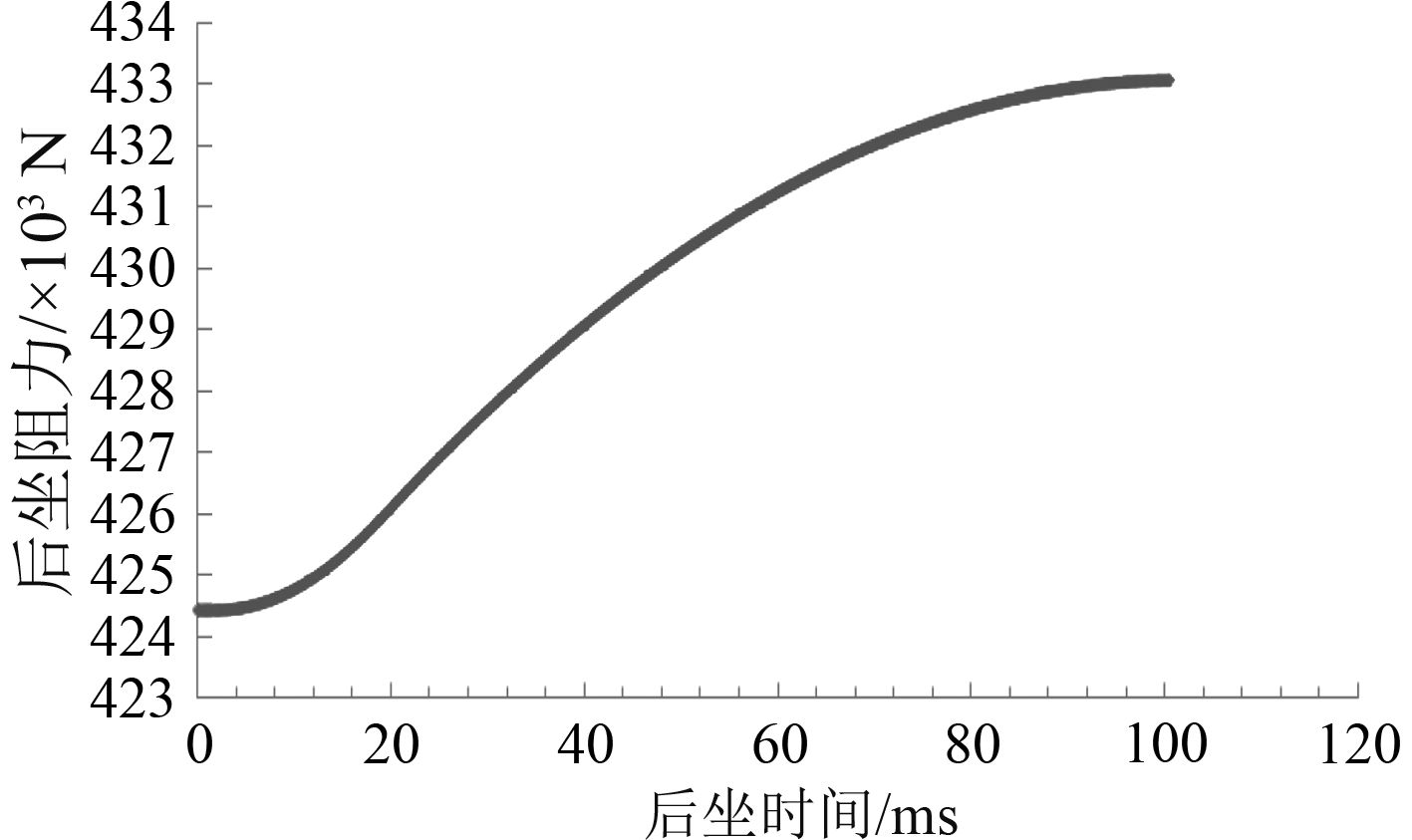图 2 后坐时间与后坐阻力曲线图 Fig. 2 The curve chart between recoil time and force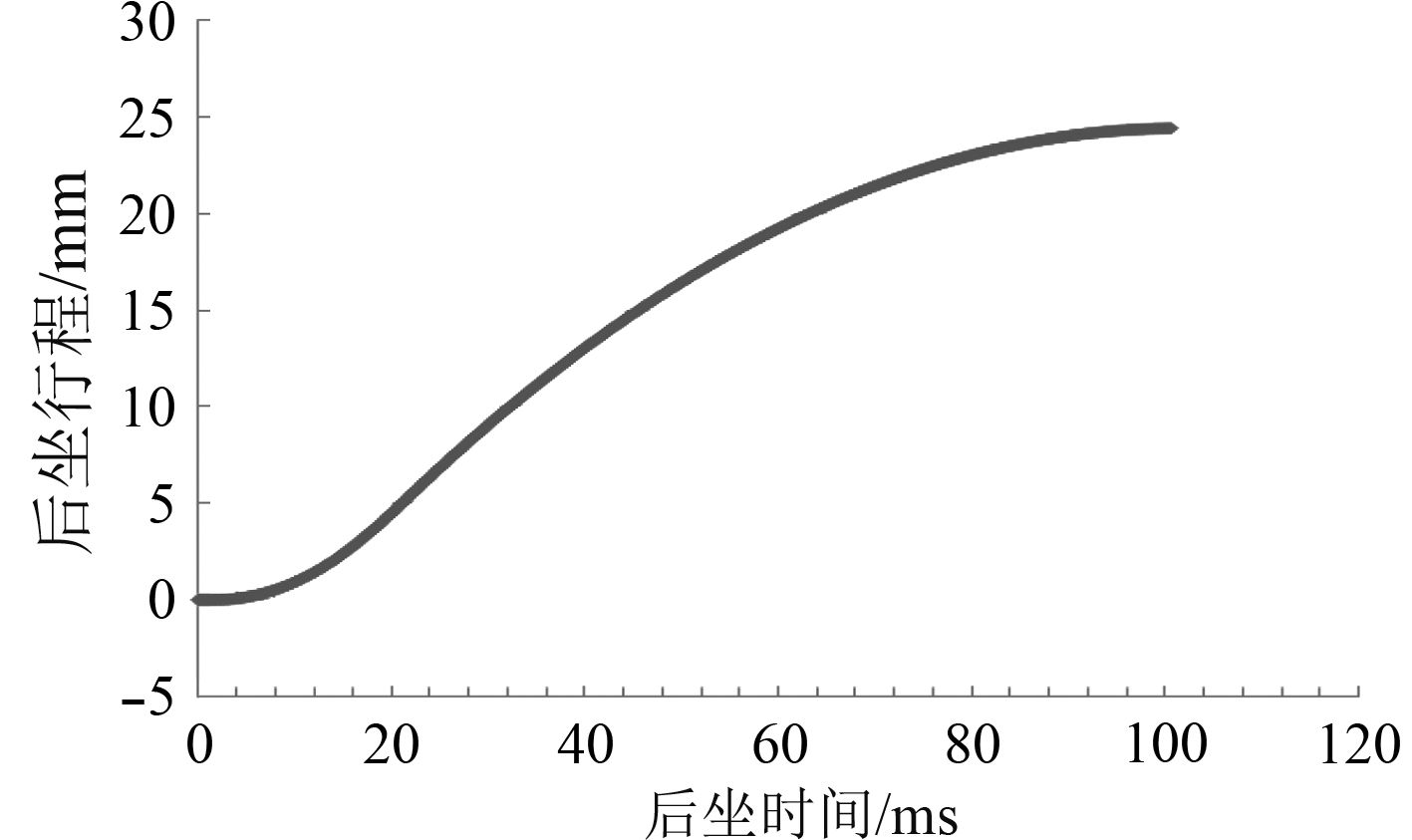图 3 后坐时间与后坐行程曲线图 Fig. 3 The curve chart between recoil time and displacement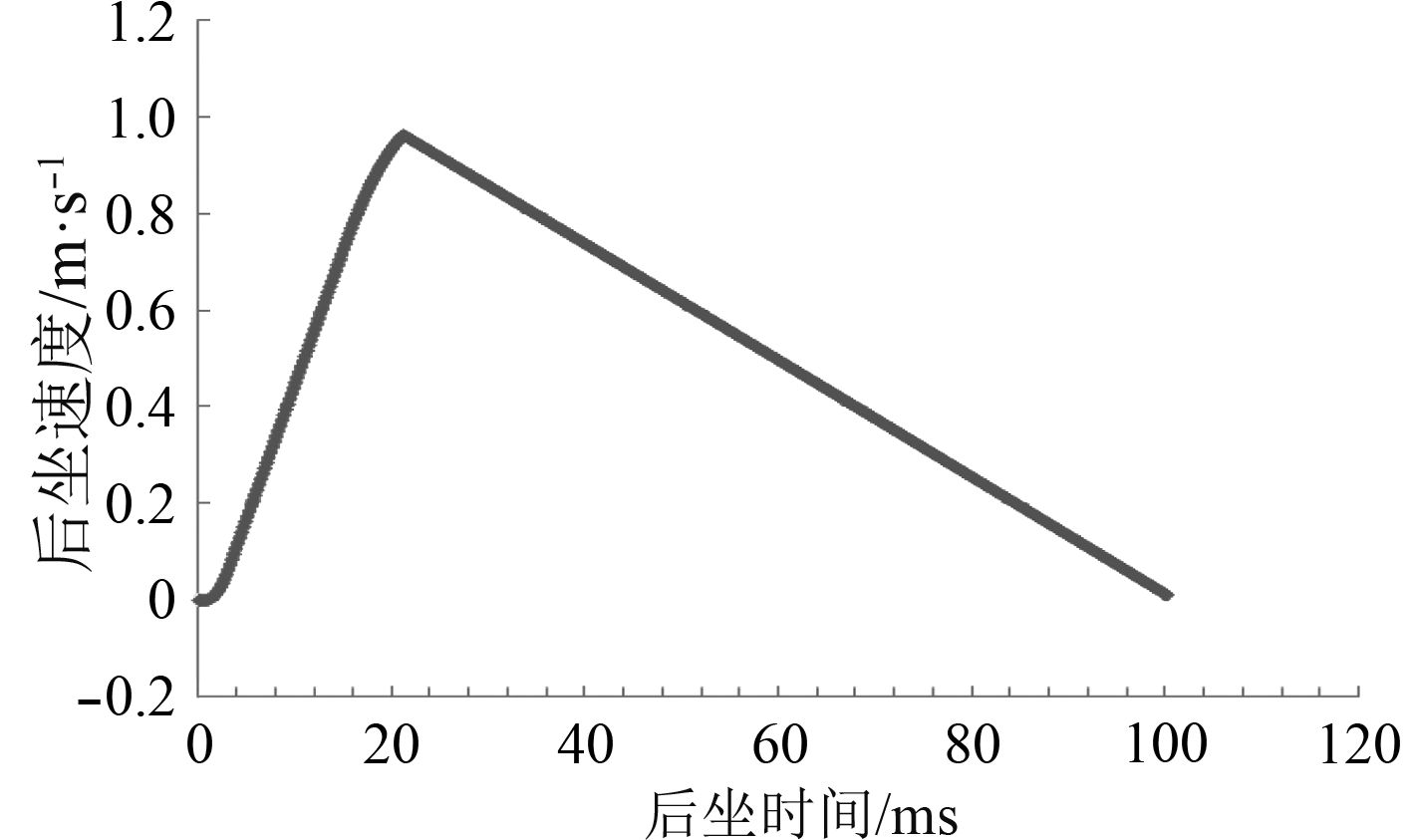图 4 后坐时间与后坐速度曲线图 Fig. 4 The curve chart between recoil time and velocity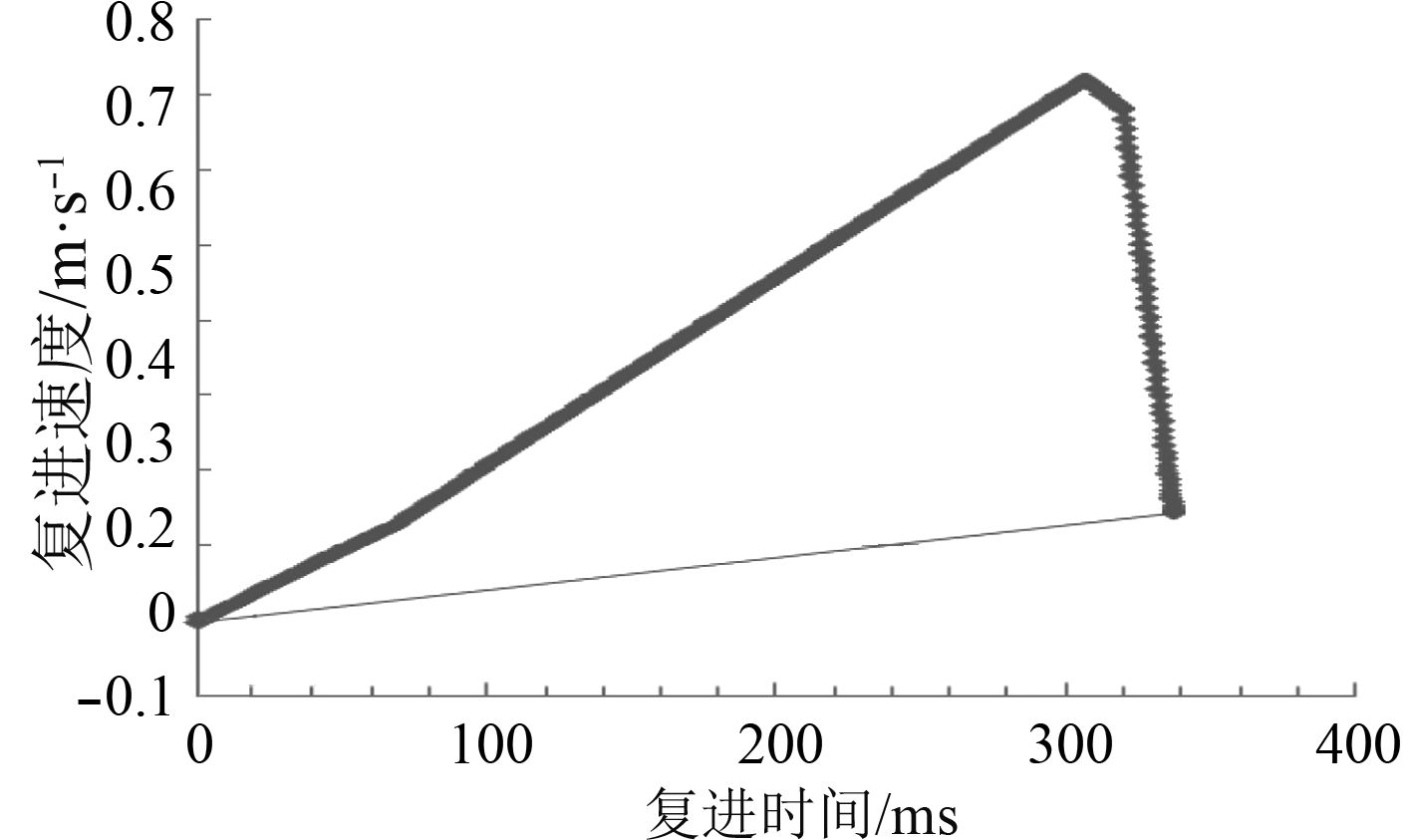图 5 复进时间与复进速度曲线图 Fig. 5 The curve chart between counter-recoil time and velocity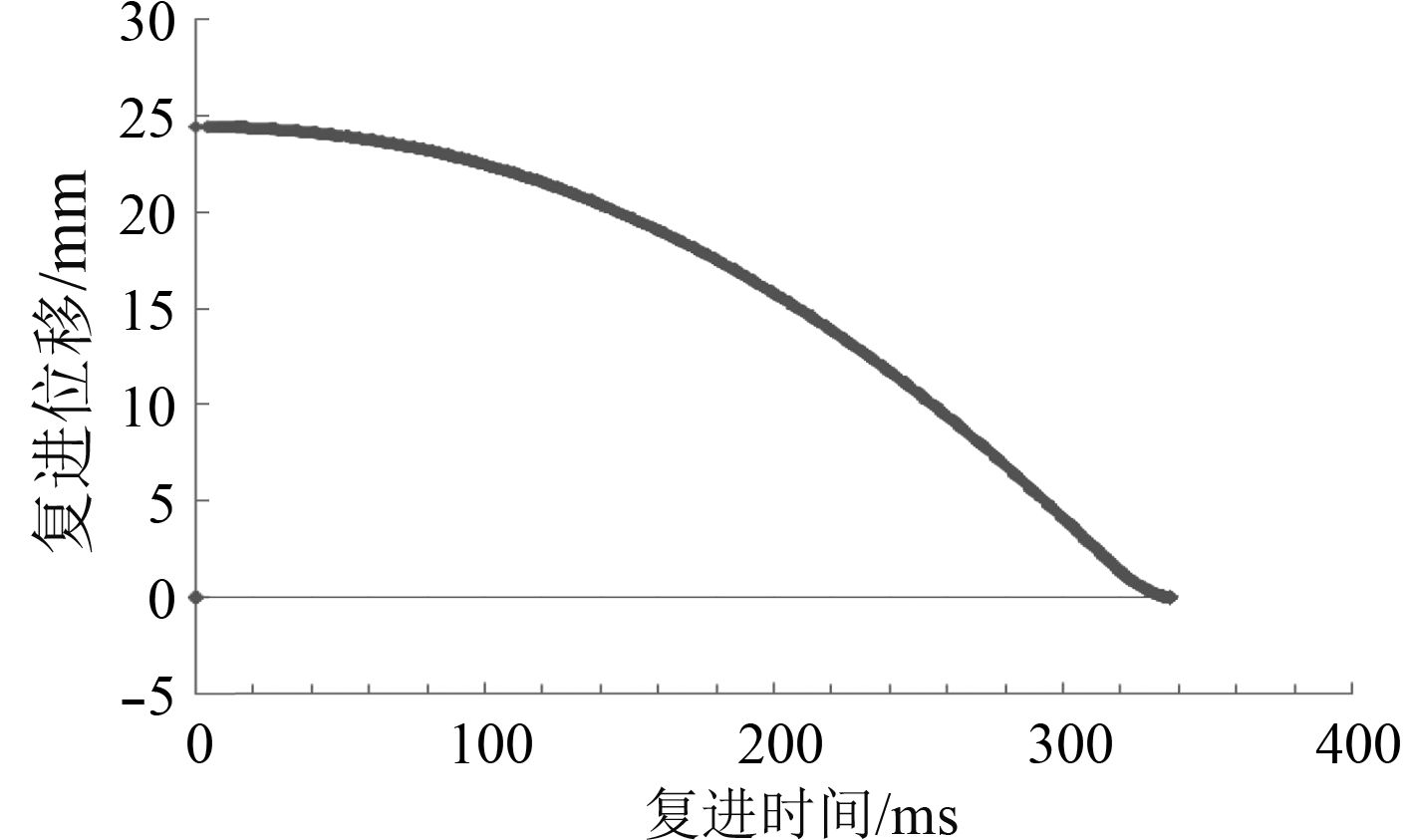图 6 复进时间与复进位移曲线图 Fig. 6 The curve chart between counter-recoil time and displacement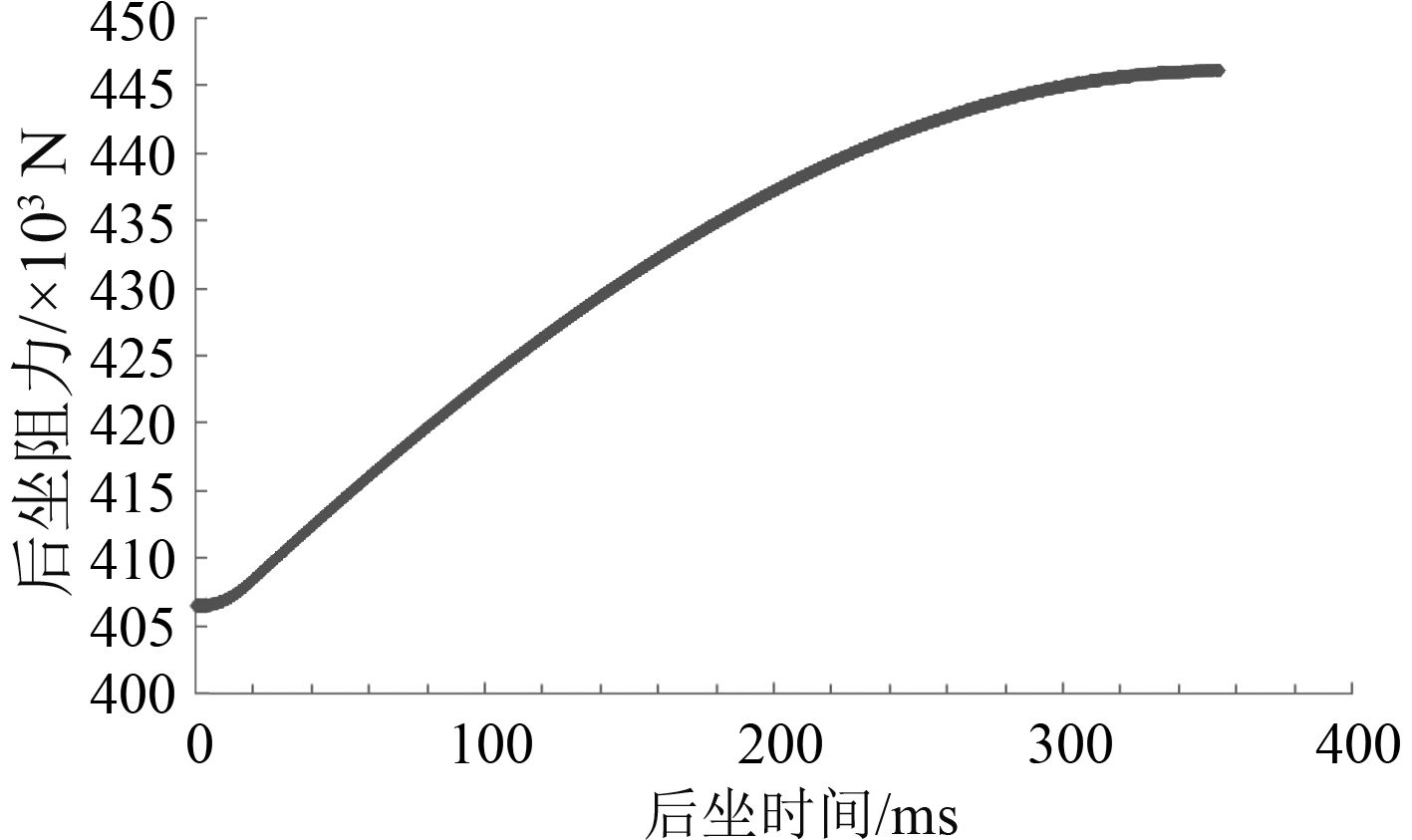图 7 后坐时间与后坐阻力曲线图 Fig. 7 The curve chart between recoil time and force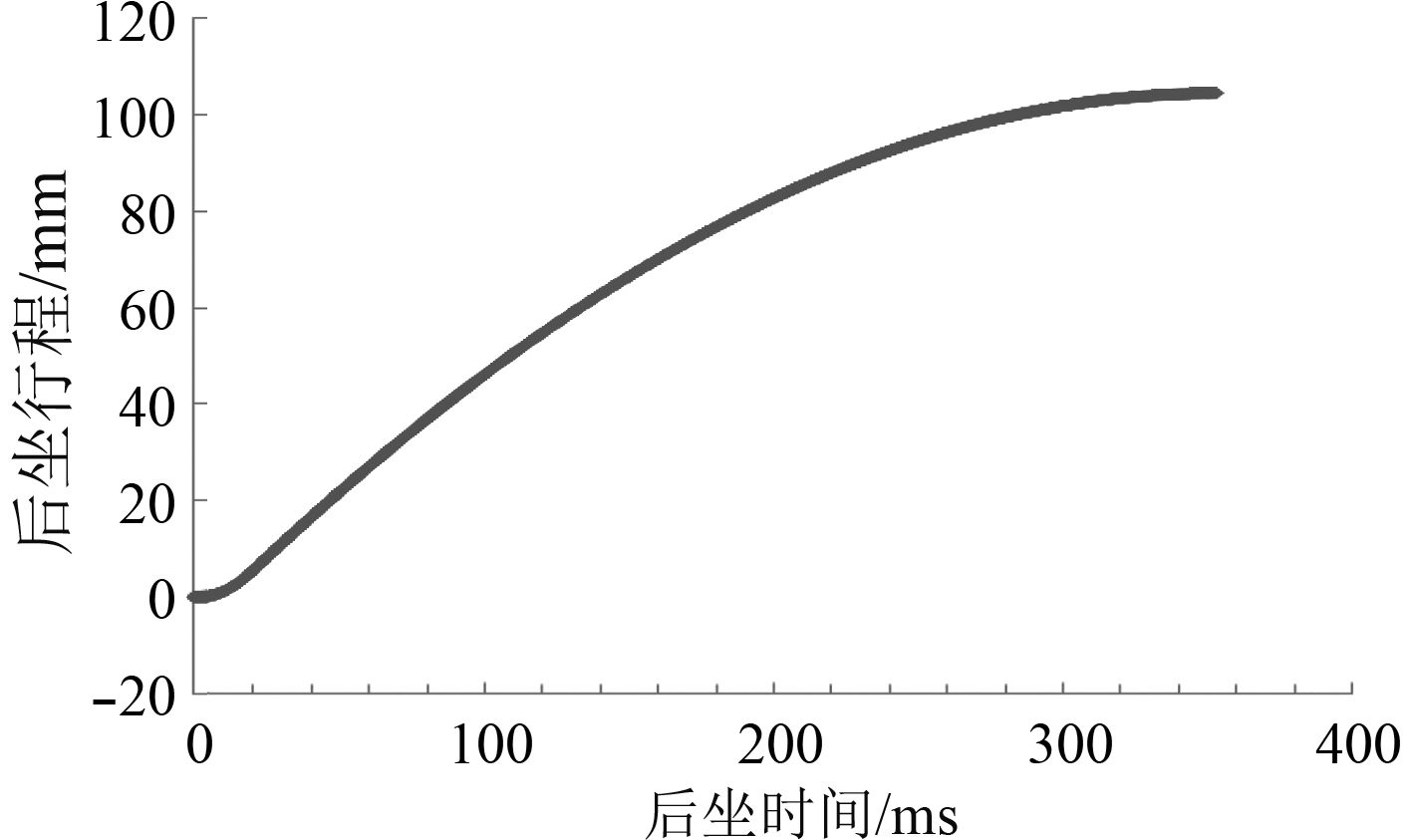图 8 后坐时间与后坐行程曲线图 Fig. 8 The curve chart between recoil time and displacement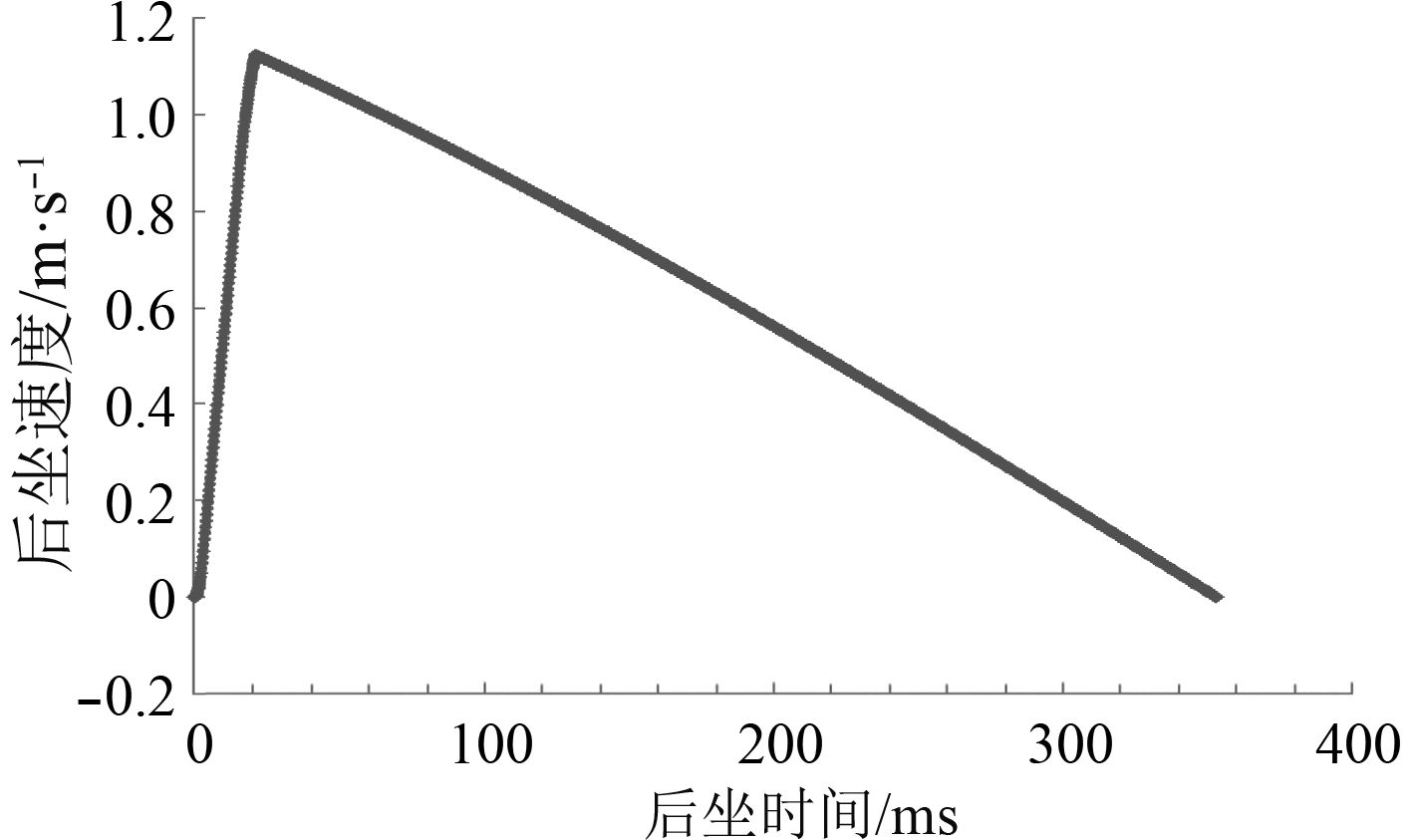图 9 后坐时间与后坐速度曲线图 Fig. 9 The curve chart between recoil time and velocity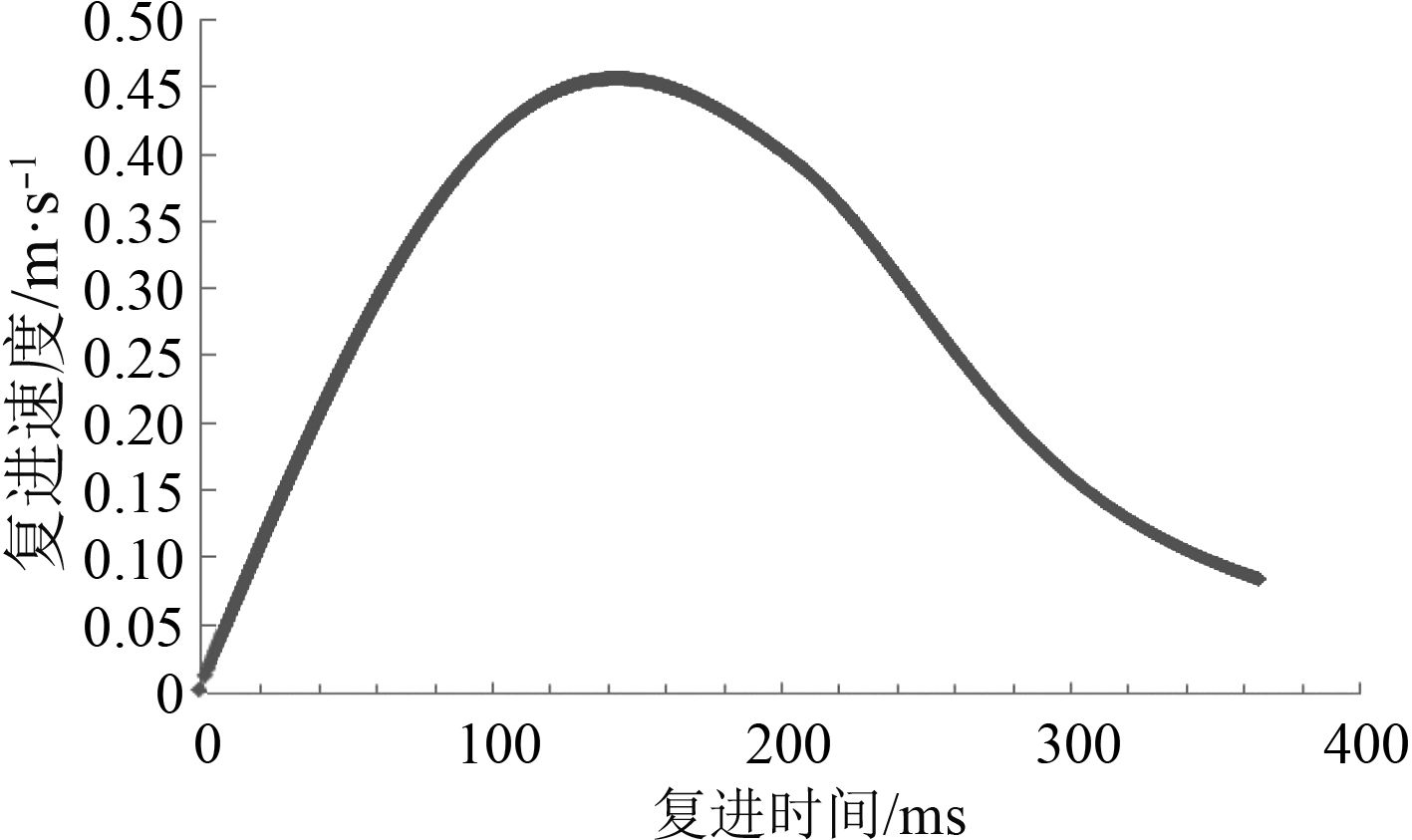图 10 复进时间与复进速度曲线图 Fig. 10 The curve chart between counter-recoil time and velocity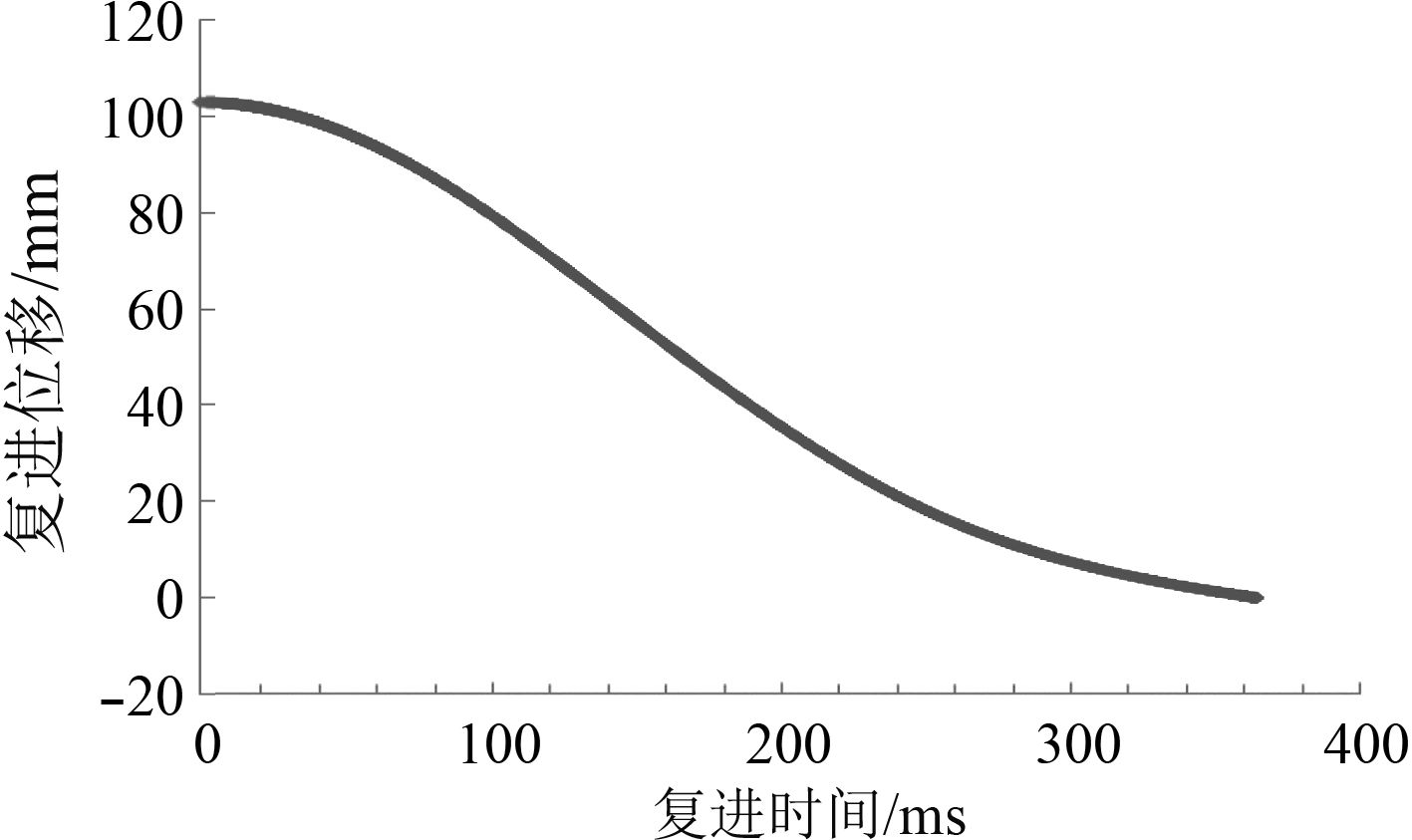图 11 复进时间与复进位移曲线图 Fig. 11 The curve chart between counter-recoil time and displacement
5 结　语

  谢克瑜, 等. 电磁轨道发射系统后坐力研究及反后坐装置设计[J]. 弹道学报, 2014, 26(4): 98-101. DOI:10.3969/j.issn.1004-499X.2014.04.019  高树滋, 陈运生, 张月林, 等. 火炮反后坐装置设计[M]. 北京: 兵器工业出版社1995.  中国国防科技信息网. 美军为电磁炮开发新型炮架, 2015. 8  ZICLINSKI A E, PARKER J V.. Demonstration of a hypervelocity mass-efficient integrated launch package[J]. IEEE Transactions on Magnetics, 2001, 37(1): 347-352. DOI:10.1109/20.911852  石江波, 栗保明. 电磁轨道炮后坐过程研究[J]. 兵工学报, 2015, 36(2): 227-233. DOI:10.3969/j.issn.1000-1093.2015.02.006